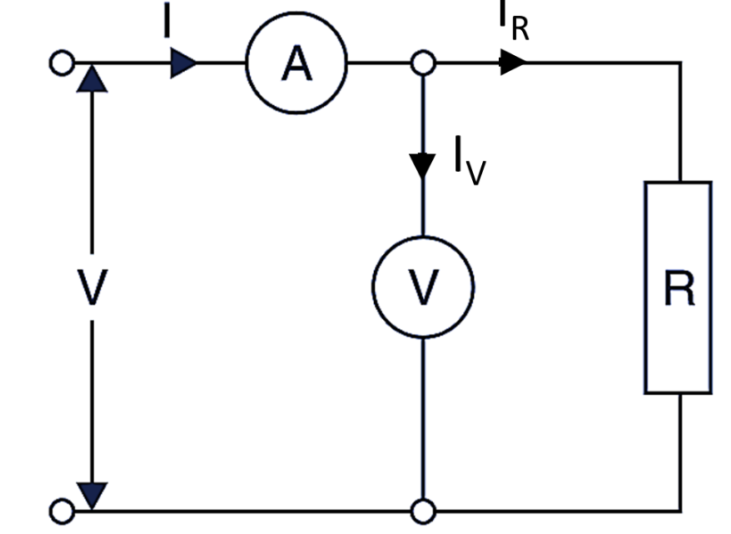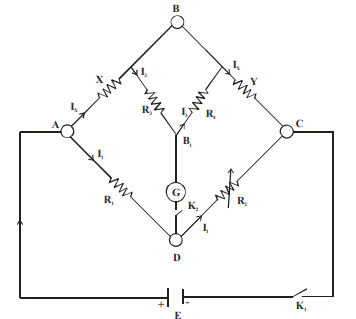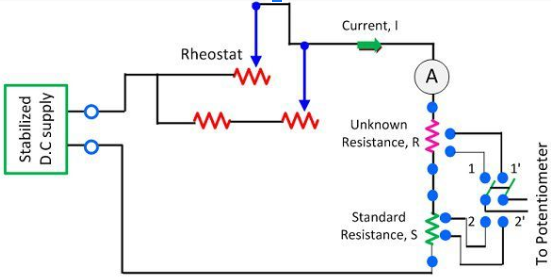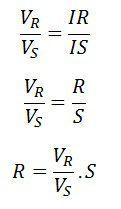## Classification of resistances

1. Low resistances: All the resistances from 1ohm and under are classified as low resistances.

2. Medium resistances: The resistance from 1Ohms  upward To about 0.1 M ohms  are included.

3. High resistances: Resistance of the order of 0.1Mohms and upward are classified as high resistances.

1. Methods For measurement of low resistances

1. Ammeter Voltmeter method

2. Kelvin Double Bridge Circuit

3. Potentiometer method

1. Ammeter Voltmeter methodIt is a simple method of measuring the lower value resistances as shown in figure the connections are made and both the current and voltage drop across the resistance simultaneously measured find the ammeter and voltmeter the accuracy mainly depends upon the accuracy and range of the instruments employed for the measurement of current and voltage.
here are current through ammeter(I) is the sum of the currents in voltmeter (Iv)And resistance(Ir), so
I=I
V+Ir
or
Ir=I-Iv
True value of of the resistance
= Voltage across the resistance
current in the resistance
=_  V___   Ohms
I-Iv

1. Kelvin Double Bridge Circuit

The Kelvin Double Bridge is one of the best devices available for the precise measurement of low resistances. It is the modification of wheatstone bridge by which the errors due to contact resistance and lead resistances are eliminated. This bridge is named double bridge because it contains a second set of ratio arms. An interesting variation of the Wheatstone bridge is the Kelvin Double Bridge, used for measuring very low resistances (typically less than 1/10 of an ohm)Let Y be the unknown and X standard known resistance of the same order.
R1, R2, R3, Rare non inductive resistances of higher values as compared to X, Y.
The Balance is obtained when the points B and D are at the same potential.
The current at A divides into I1 and Ix in arms R1 and X respectively and I3 passes through R3, Potential drop on AD arm = I1R1
The sum of potential drops across X and R3 = Ix X + I3R3 But potential at B and D are the same for no deflection in galvanometer, which implies that –
I1R1 = Ix X + I3R3 ………………….. (1)
Similarly,
P.D. across R2 = P.D. across R4 + P.D. across Y
or
I1R2 = I3R4 + Ix Y ………………… (2)
From (1) and (2)
we get Ix = I1R1 – I3R3 Ix y = I1R2 – I3R4 Dividing

OR

But

........................(3)

This equation gives the value of Y in terms of known X1R1, R2 resistances.

1. Measurement of Resistance using Potentiometer

The DC potentiometer method of measurement of resistance is used for measuring the unknown resistance of low value. This can be done by comparing the unknown resistance with the standard resistance. The voltage drop across the known and unknown resistance is measured and by comparison the value of known resistance is determined.
Following is the  circuit diagram of potentiometer where R is the unknown resistance whose value needs to be measured. The S is the standard resistance from which the value of unknown resistance is compared. The rheostat is used for controlling the magnitude of current into the circuit.• Measurement of Resistance

The double pole double throw switch is used in the circuit. The switch, when moved to position 1, 1  the unknown resistance connects to the circuit, and when it moves to position 2, 2 the standard resistance connects to the circuit.
Consider that when the switch is in position 1,1 the voltage drop across the unknown resistance is Vr
Vr=IR        (1)
and when it is in 2, 2 the voltage drop across the resistance is Vs
Vs=IS    (2)
On equating the equation (1) and (2), we getThe accuracy of unknown resistance depends on the value of standard resistance.The accuracy of the unknown resistance also depends on the magnitude of the current at the time of the readings. If the magnitude of current remains the same, the circuit gives the accurate result. The ammeter is used in the circuit for determining the magnitude of current passing through the resistor during the reading.The magnitude of the current is adjusted in such a way that the voltage drop across the resistance is equal to 1 volt.